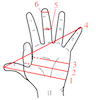#### You may also likeWhich units would you choose best to fit these situations?

# Bigger or Smaller?

##### Age 14 to 16Challenge Level

When measuring some property of an object the number we measure depends on the units chosen. For example, 1cm = 0.01m, so converting from cm to m makes the number get smaller; we need more small units to make up the number of big units. In each case below, does the number get bigger or smaller following a change in units? Can you estimate without a calculator an approximate factor by which the numbers would change in each case?

1. 1 cm $^2\rightarrow ??$ m $^2$
2. 1 foot $\rightarrow ??$ inches
3. 1 mile $\rightarrow ??$ kilometers
4. 1 litre $\rightarrow ??$ cm $^3$
5. 1 foot $^3\rightarrow ??$ inches $^3$
6. 1 m s $^{-1}\rightarrow ??$ miles / hour
7. 1 mm $^3\rightarrow ??$ m $^3$
8. 1 degrees C $\rightarrow ??$ degrees K
9. 85 degrees $\rightarrow ??$ radians
10. 1 Pa $\rightarrow ??$ cm$^{-1}$ g s$^{-2}$
11. 1 W $\rightarrow ??$ cm$^2$ g s$^{-3}$
12. 1 Hz $\rightarrow ??$ per minute
13. 1 Mol $\rightarrow ??$ trillion
14. Molarity of 1$\rightarrow ??$ per cm$^3$
15. 1 katal $\rightarrow ??$ million million per picosecond

Can you make up some of your own similar problems?

Other problems

Try the fun Zin Obelisk task from the main NRICH site